Download Presentation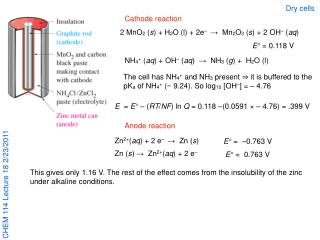CHEM 114 Lecture 18 2/23/2011

# CHEM 114 Lecture 18 2/23/2011 - PowerPoint PPT Presentation

Dry cells. Cathode reaction. 2 MnO 2 ( s ) + H 2 O (l) + 2e – → Mn 2 O 3 ( s ) + 2 OH – ( aq ). E° = 0.118 V. NH 4 + ( aq ) + OH – ( aq ) → NH 3 ( g ) + H 2 O (l).I am the owner, or an agent authorized to act on behalf of the owner, of the copyrighted work described.
Download Presentation## CHEM 114 Lecture 18 2/23/2011

An Image/Link below is provided (as is) to download presentation

Download Policy: Content on the Website is provided to you AS IS for your information and personal use and may not be sold / licensed / shared on other websites without getting consent from its author.While downloading, if for some reason you are not able to download a presentation, the publisher may have deleted the file from their server.

- - - - - - - - - - - - - - - - - - - - - - - - - - E N D - - - - - - - - - - - - - - - - - - - - - - - - - -
Presentation Transcript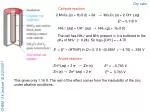Dry cells

Cathode reaction

2 MnO2 (s) + H2O (l) + 2e– → Mn2O3 (s) + 2 OH– (aq)

E° = 0.118 V

NH4+ (aq) + OH– (aq) → NH3 (g) + H2O (l)

The cell has NH4+ and NH3 present ⇒ it is buffered to the pKa of NH4+ (~ 9.24). So log10 [OH–] = – 4.76

E = E° – (RT/NF) ln Q = 0.118 –(0.0591 × – 4.76) = .399 V

Anode reaction

E° = –0.763 V

Zn2+(aq) + 2 e– → Zn (s)

E° = 0.763 V

Zn (s) → Zn2+(aq) + 2 e–

This gives only 1.16 V. The rest of the effect comes from the insolubility of the zinc under alkaline conditions.

CHEM 114 Lecture 18 2/23/2011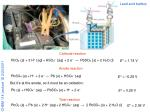Cathode reaction

E° = 1.74 V

PbO2 (s) + 3 H+ (aq)+HSO4– (aq) + 2 e– → PbSO4 (s) + 2 H2O (l)

Anode reaction

E° = –0.28 V

PbSO4 (s)+ H+ + 2 e– → Pb (s) + HSO4– (aq)

But it’s at the anode, so it must be an oxidation:

CHEM 114 Lecture 18 2/23/2011

E° = 0.28 V

Pb (s) + HSO4– (aq) → PbSO4 (s)+ H+ + 2 e–

Total reaction

E° = 2.06 V

PbO2 (s) + Pb (s) + 2 H+ (aq)+2HSO4– (aq) → 2 PbSO4 (s) + 2 H2O (l)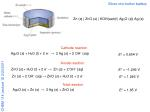Silver-zinc button battery

Zn (s) | ZnO (s) | KOH(satd) | Ag2O (s)| Ag (s)

Cathode reaction

Ag2O (s) + H2O (l) + 2 e– → 2 Ag (s) + 2 OH– (aq)

E° = 0.604 V

Anode reaction

E° = –1.246 V

ZnO (s)+ H2O (l) + 2 e– → Zn (s) + 2 OH– (aq)

E° = 1.246 V

Zn (s) + 2 OH– (aq) → ZnO (s)+ H2O (l) + 2 e–

Total reaction

CHEM 114 Lecture 18 2/23/2011

E° = 1.85 V

Ag2O (s) + Zn (s) → 2 Ag (s) + ZnO (s)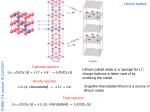Lithium battery

Cathode reaction

Lithium cobalt oxide is a ‘sponge for Li+; charge balance is taken care of by oxidizing the cobalt.

Li(1–x)CoO2 (s) + x Li+ + x e– → LiCoO2 (s)

Anode reaction

CHEM 114 Lecture 18 2/23/2011

Graphite-intercalated lithium is a source of lithium metal

x Li (s, intercalated)→ x Li+ + x e–

Total reaction

Li(1–x)CoO2 (s) + x Li (s, intercalated) → LiCoO2 (s)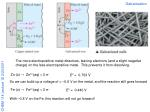Galvanization

The more electropositive metal dissolves, leaving electrons (and a slight negative charge) on the less electropositive metal. This prevents it from dissolving.

E° = 0.763 V

Zn (s) → Zn2+(aq) + 2 e–

So we can build up a voltage of > –0.8 V on the metal, and the reaction still goes forward

CHEM 114 Lecture 18 2/23/2011

E° = 0.440 V

Fe (s) → Fe2+(aq) + 2 e–

With –0.8 V on the Fe, this reaction will not go forward!Sacrificial anodes

CHEM 114 Lecture 18 2/23/2011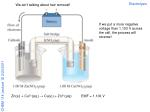Electrolysis

We ain’t talking about hair removal!

If we put a more negative voltage than 1.103 V across the cell, the process will reverse!

CHEM 114 Lecture 18 2/23/2011

Zn(s.) + Cu2+(aq.) → Cu(s.) + Zn2+(aq.)

EMF = 1.103 V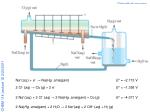Chloralkali process

Na+(aq.) + e– → Na(Hg. amalgam)

E° = –2.713 V

CHEM 114 Lecture 18 2/23/2011

2 Cl– (aq) → Cl2 (g) + 2 e–

E° = –1.358 V

2 Na+(aq.) + 2 Cl– (aq) → 2 Na(Hg. amalgam) + Cl2 (g)

E° = –4.071 V

2 Na(Hg. amalgam) + 2 H2O → 2 Na+(aq) + 2 OH– (aq) + H2 (g)